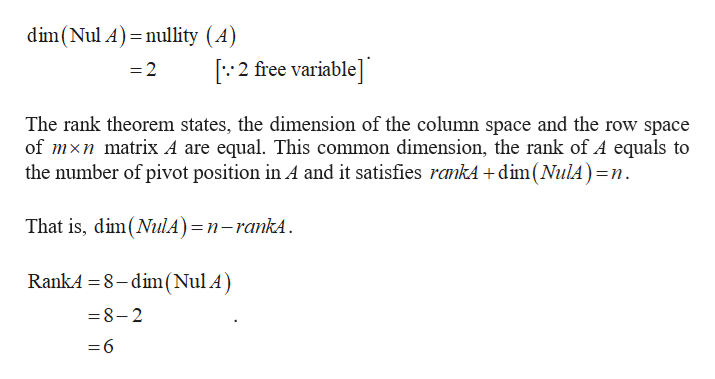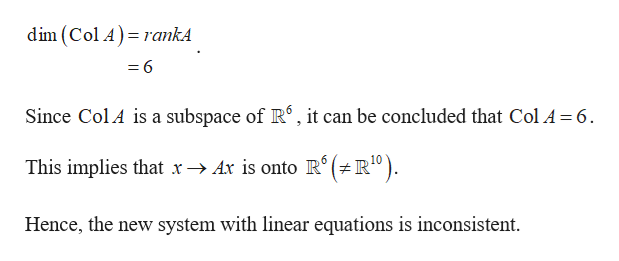# Hello,I am not confident in this Linear Algebra question: Suppose that a nonhomogeneous system with 10 linear equations in 8 unknowns has a solution with 2 free variables. Is it possible to change some constants on the equations’ right hand side to make the new system inconsistent? Explain in detail. Help would be greatly appreciated

Question

Hello,

I am not confident in this Linear Algebra question:

Suppose that a nonhomogeneous system with 10 linear equations in 8 unknowns has a solution with 2 free variables. Is it possible to change some constants on the equations’ right hand side to make the new system inconsistent? Explain in detail.

Help would be greatly appreciated

check_circleExpert Solution
Step 1

Consider the system,

Step 2

It is given that two free variable.help_outlineImage Transcriptionclosedim(Nul A) nullity (A) 2 free variable] =2 The rank theorem states, the dimension of the column space and the row space of mxn matrix A are equal. This common dimension, the rank of A equals to the number of pivot position in A and it satisfies rankA+dim(NulA)=n That is, dim(NulA)=n-rankA RankA 8-dim(Nul A) 8-2 = 6 fullscreen
Step 3

Thus, the dimension of column...help_outlineImage Transcriptionclosedim (Col A) rankA - 6 Since ColA is a subspace of R, it can be concluded that Col A = 6 (R"). 6 10 This implies that x-> Ax is onto R° Hence, the new system with linear equations is inconsistent fullscreen

### Want to see the full answer?

See Solution

#### Want to see this answer and more?

Solutions are written by subject experts who are available 24/7. Questions are typically answered within 1 hour*

See Solution
*Response times may vary by subject and question
Tagged in

### Math